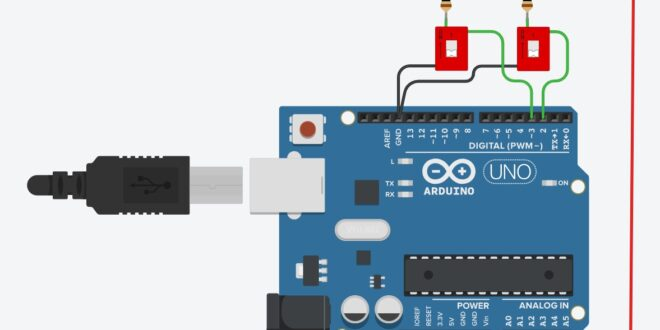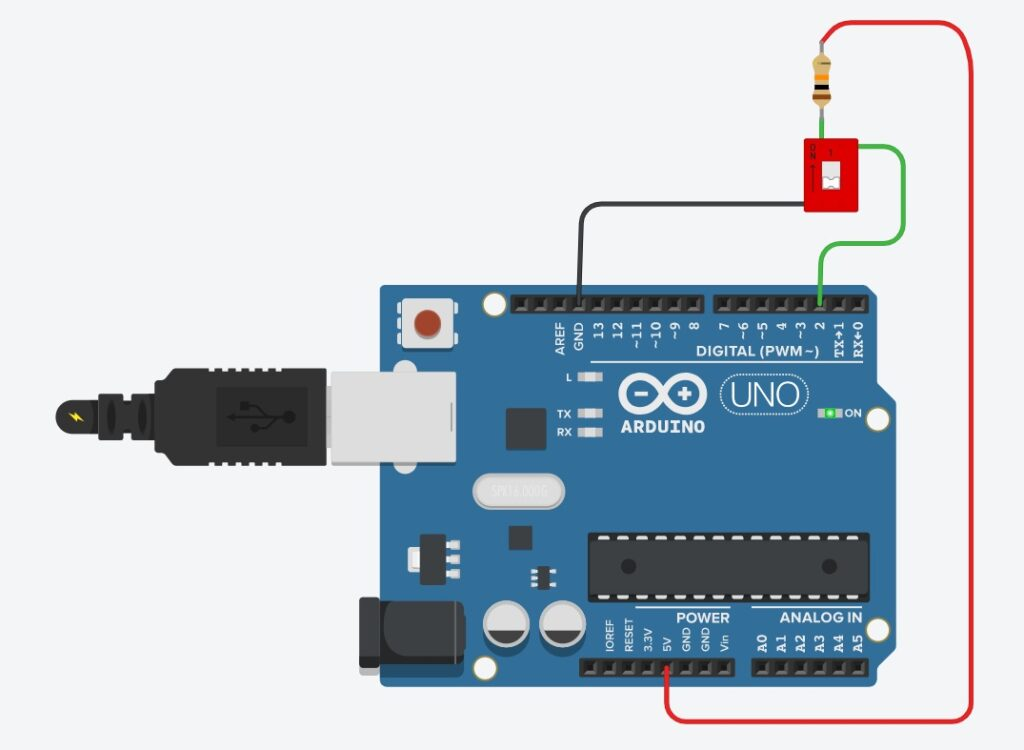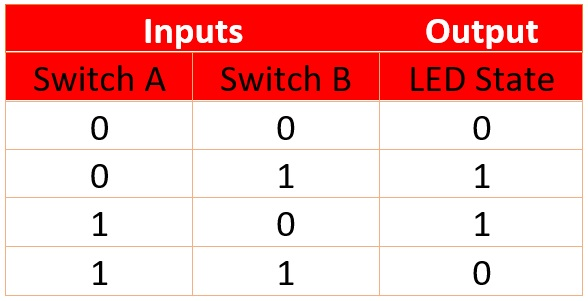Home / Tutorials / Arduino Tutorial / Arduino Programming: Ternary Operator# Arduino Programming: Ternary Operator

If your Arduino code involves decision-making, then it's very likely you're using conditional statements. Using if ... else... is common;  switch statements as well. But there is a third way, using ternary operator, that will really make your code shorter.

### Example Scenario

Say we are reading the state of an (active low) switch, on pin 2 of an Arduino. The switch controls a LED on pin 13, that is if the switch is down, the LED turns on, and so on. The circuit is shown in the next section.

The if ... else... statement for this scenario would be like this:

```if(digitalRead(2) ==  LOW){
digitalWrite(13, HIGH);
}else{
digitalWrite(13, LOW);
}```

We can actually make this block a single line of code!

### Ternary Operator

The ternary operator in Arduino programming is the "?" operator and is used like this:

`expressionA ? expressionB : expressionC`

The way to read it is "is expressionA true? if it is then execute expressionB, otherwise, execute expressionC".

Going back to our scenario, the ternary operator equivalent of the if...else... statement is:

`digitalRead(2) ? digitalWrite(13, LOW) : digitalWrite(13, HIGH);`

Here, digitalRead(2) returns either LOW or HIGH which are equivalent to logic FALSE or TRUE respectively. Hence, if the switch is closed, then digitalRead(2) returns FALSE, and digitalWrite(13, HIGH) is executed. Otherwise, the LED is turned off.You can also use the ternary operator to quickly assign a value to a variable given a condition. For example, you have a temperature sensor on analog pin 0. But you only need to acquire the sensor value if it's greater than a certain amount, say, 50 degrees. The code will now be:

`int acq_temp = (sensor_temp > 50) ? sensor_temp : 0;`

Here, acq_temp will have a value equal to sensor_temp if it's greater than 50, otherwise, acq_temp will be zero. This assumes that sensor_temp is another int variable.

### Nested Ternary Operators

Ternary operators can also be nested and will be equivalent to an if...else-if...else... statement. Say we want to implement the logic XOR function using two switches on an Arduino.

The XOR function has a truth table:Basically, the LED should only turn on when only one of the switches is closed. Other than that, the LED stays off.

We attach the second switch to pin 3 of the Arduino while the switch on pin 2 and the LED on pin 13 remains.The statement would now be:

`digitalRead(2) ? (digitalRead(3) ? digitalWrite(13, LOW) : digitalWrite(13, HIGH)): (digitalRead(3) ? digitalWrite(13, HIGH) : digitalWrite(13, LOW));`

The first part of this statement checks the state of pin 2. If it's HIGH, then it checks the state of pin 3. When both pin 2 and pin 3 are high, then the LED turns off. If pin 3 is LOW, the LED stays off. If however pin 2 is LOW from the first check, it checks pin 3 again if it's HIGH or LOW. If it's the latter, then the LED is off. Otherwise, the LED turns on.

The statement is equivalent to:

```if (digitalRead(2) == LOW){ //2 is low
if (digitalRead(3) == HIGH){ //2 is low 3 is high
digitalWrite(13, HIGH);
}else{
digitalWrite(13, LOW); //2 is low 3 is low
}
}else{ //2 is high
if (digitalRead(3) == LOW){ //2 is high 3 is low
digitalWrite(13, HIGH);
}else{
digitalWrite(13, LOW); //2 is high 3 is high
}
}

```